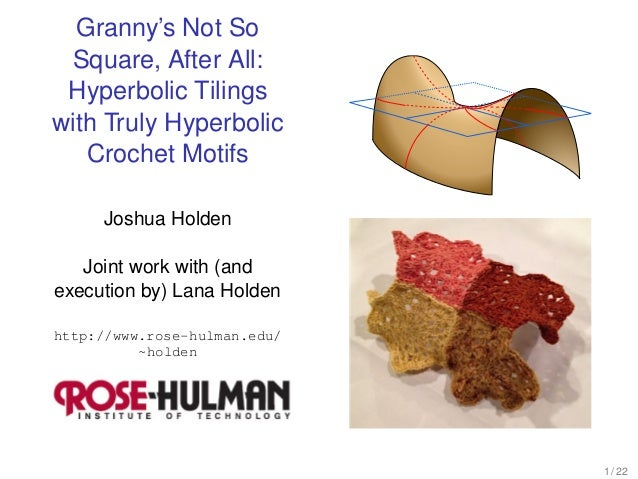Successfully reported this slideshow.
We use your LinkedIn profile and activity data to personalize ads and to show you more relevant ads. You can change your ad preferences anytime.Upcoming SlideShare
×

Granny’s Not So Square, After All: Hyperbolic Tilings with Truly Hyperbolic Crochet Motifs

1,630 views

Published on

Until now, most methods for making a hyperbolic plane from crochet or similar fabrics have fallen into one of two categories. In one type, the work starts from a point or line and expands in a sequence of increasingly long rows, creating a constant negative curvature. In the other, polygonal tiles are created out of a more or less Euclidean fabric and then attached in such a way that the final product approximates a hyperbolic plane on the large scale with an average negative curvature. On the small scale, however, the curvature of the fabric will be closer to zero near the center of the tiles and more negative near the vertices and edges, depending on the amount of stretch in the fabric. The goal of this project is to show how crochet can be used to create polygonal tiles which themselves have constant negative curvature and can therefore be joined into a large region of a hyperbolic plane without significant stretching. Formulas from hyperbolic trigonometry are used to show how, in theory, any regular tiling of the hyperbolic plane can be produced in this way.

Published in: Education
• Full Name
Comment goes here.

Are you sure you want to Yes No
Your message goes here• Be the first to comment

Granny’s Not So Square, After All: Hyperbolic Tilings with Truly Hyperbolic Crochet Motifs

1. 1. Granny’s Not So Square, After All: Hyperbolic Tilings with Truly Hyperbolic Crochet Motifs Joshua Holden Joint work with (and execution by) Lana Holden http://www.rose-hulman.edu/ ~holden 1 / 22
2. 2. A hyperbolic plane is a surface with constant negative curvature. [The Geometry Center] 2 / 22
3. 3. A positive curvature surface bends away from the same side of its tangent plane in every direction. [Wikipedia] 3 / 22
4. 4. A zero curvature surface is ﬂat (in at least one direction). [Robert Gardner, ETSU] 4 / 22
5. 5. A negative curvature surface bends away from different sides of its tangent plane. [Wikipedia] 5 / 22
6. 6. Also, on a negative curvature surface circles have larger circumferences than they “should”. (This is the Bertrand-Diquet-Puiseux Theorem.) [Daina Taimina and the Institute For Figuring] 6 / 22
7. 7. So one way to produce a hyperbolic plane is to construct it in arcs of exponentially increasing length. [Daina Taimina and the Institute For Figuring] Daina Taimina realized that you could do this with crochet stitches. 7 / 22
8. 8. A regular tiling ﬁlls a surface completely with congruent regular polygons. [Lana Holden] 8 / 22
9. 9. A regular tiling of the hyperbolic plane has more polygons around each vertex than it “should”. [Wikipedia] And correspondingly, the interior angles are smaller than they “should be”. 9 / 22
10. 10. We could construct the surface ﬁrst and then tile it. [Daina Taimina] 10 / 22
11. 11. Or we could make ﬂat tiles and attach them in such a way that they curve negatively. [Helaman Ferguson and Jeffrey Weeks] Helaman Ferguson did this with stretchy materials such as polar ﬂeece that distribute the curvature. 11 / 22
12. 12. Our goal is to make tiles which are the correct shape and the correct curvature. [Lana Holden] (Daina Taimina previously made some progress towards this.) 12 / 22
13. 13. To calculate the correct shape, we use the (Second) Hyperbolic Law of Cosines: [Wikipedia] cos C = − cos A cos B + sin A sin B cosh c 13 / 22
14. 14. The number of sides of the polygon and the number of polygons around a vertex determine the angles. For our construction, we need to know the inradius, the circumradius, and the side length. 14 / 22
15. 15. We will crochet the tiles using variations of the classic “granny square”. [Purl Soho and purlbee.com] The inradius determines the number of rounds. 15 / 22
16. 16. We need to vary the pattern to add the exponentially increasing length. We add exponentially spaced increases to achieve the desired side length. 16 / 22
17. 17. We need to vary the pattern to add the exponentially increasing length. We substitute longer stitches to achieve the desired circumradius. 17 / 22
18. 18. Et voilà! [Lana Holden] Five of these hyperbolic squares go around each vertex, rather than the “usual” four. 18 / 22
19. 19. Other “granny polygons” are also found in modern crochet. [Lana Holden] Here we have put three “granny hexagons” around each vertex. 19 / 22
20. 20. And we can make hyperbolic versions of some of them. [Lana Holden] Here we have put four “granny hexagons” around each vertex. 20 / 22
21. 21. In theory we could construct any hyperbolic tiling. However, as the interior angles get sharper, it will become more and more difﬁcult to turn the corners. 21 / 22
22. 22. Hope you enjoyed the show! 22 / 22